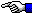No Active Partition Found
(Pathways through the Windows 7 MBR)

If you need any help in setting up the Bochs Debugger, please email us.Back to: Pathways through the Windows 7 MBR page.

When each of the four 16-byte Partition Table entries (there can only be 4 entries under the standard 'Basic Disk'/MBR Partitioning Scheme) has only zero (00 hex) as its first byte; that is, no Active partition is found, the PC's BIOS will automatically jump to Interrupt 18h (often labeled as "ROM BASIC" since the original IBM® PC's XT and AT models contained the BASIC Programming Language in ROM chips). Starting back at instruction 0x0623, we show a 00 byte at Memory location 0x07BE:

 ```The code just put 07be into the Base Pointer (BP) Register to check for 80h at the correct offset in the first Partition Table entry: AX=0000 BX=0000 CX=0004 DX=0000 SP=7C00 BP=07BE SI=7E00 DI=0800 DS=0000 ES=0000 SS=0000 CS=0000 IP=0623 of df IF sf ZF af PF cf NV UP EI PL ZR NA PE NC 0623 807E0000 CMP BYTE PTR [BP+00],00 SS:07BE=00```

Since we're comparing zero with zero, the result of the CMP instruction is also zero, and the Zero and Parity flags both remain set (ZF=1; PF=1); with no change in the Sign flag either (sf=0). So when we execute the JL (Jump if Less than zero) instruction:

 ```AX=0000 BX=0000 CX=0004 DX=0000 SP=7C00 BP=07BE SI=7E00 DI=0800 DS=0000 ES=0000 SS=0000 CS=0000 IP=0627 of df IF sf ZF af PF cf NV UP EI PL ZR NA PE NC 0627 7C0B JL 0634```

More will be posted in the near future... this page is still Under Construction !!!

If you need any help in setting up the Bochs Debugger, please email us.

Date Page Added: July 5, 2013 (05.07.2013).

You can write to us using this: online reply form. (It opens in a new window.)Pathways through the Windows 7 MBRThe Starman's x86 ASSEMBLY PagesThe Starman's Realm Index Page